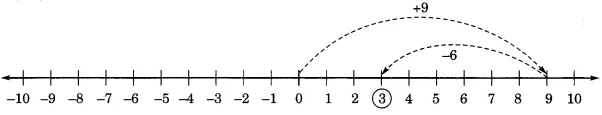# NCERT Solutions for Class 6 Maths Chapter 6 Integers

NCERT solutions for class 6 maths chapter 6 Integers- You have studied about whole numbers and natural numbers in the second chapter of this class. Now we are going to study integers in this chapter. Before discussing the integers you must have cleared concepts regarding natural numbers and whole numbers. CBSE NCERT solutions for class 6 maths chapter 6 Integers is covering the solutions of each question of the integer. Natural numbers are the collection of 1, 2, 3, 4, 5,..... If you include number 0 (zero) to this collection you get a new collection of numbers known as whole numbers. So, the whole number is the collection of 0, 1, 2, 3, 4,... You have studied these numbers in the second chapter. If you put the negative numbers and the whole numbers together, the new collection of numbers will look like –1, – 2, – 3,–4,  –5,...... 0, 1, 2, 3, 4, 5,..., and this collection of numbers is known as Integers. Integers are the collection of numbers between negative infinity to positive infinity ( – 3, – 2, – 1, 0, 1, 2, 3). Numbers 1, 2, 3,.... called positive numbers are the positive integers and – 1, – 2, – 3, ... called negative integers. Solutions of NCERT for class 6 maths chapter 6 Integers are there to assist you while solving the unsolved problems. This chapter contains a total of 19 questions in 3 exercises. NCERT solutions for class 6 maths chapter 6 Integers is covering every question in detail. If you need NCERT Solutions for other classes and subjects then you click on the given link. All the exercises are listed below.

Exercise 6.1

Exercise 6.2

Exercise 6.3

NCERT solutions for class 6 maths chapter 6 Integers Exercise: 6.1

(a) Increase in weight

(b)  north

(c) east

(d) Loss of

(e) above sea level

The opposites are-

(a) Decrease in weight

(b) 30 km south

(d) profit of Rs 700

(e) 100 m below sea level

(a) An airplane is flying at a height two thousand meters above the ground.

(b) A submarine is moving at a depth, eight hundred metre below the sea level.

(c) A deposit of rupees two hundred.

(d) Withdrawal of rupees seven hundred.

(a) An airplane is flying at a height two thousand meters above the ground. So it can be written as-
+2000m

(b) A submarine is moving at a depth, eight hundred meters below the sea level. In integers, it can be written as-
- 800m

(c) A deposit of rupees two hundred. In integer form, it can be written as-

+ Rs 200

(d) Withdrawal of rupees seven hundred. It can be written as-

- Rs 700

(a) + 5

(b) – 10

(c) + 8

(d) – 1

(e) – 6

Draw a straight line and place a mark on it at a distance of the 1cm unit.

(a) Here A indicates the position of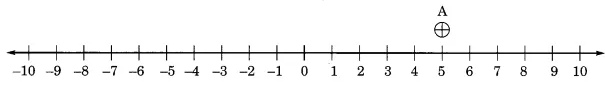(b) Here B indicates the position of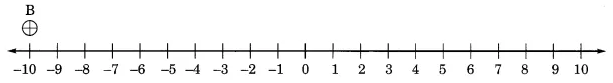(c) Here C represents the position of(d) Here D represents the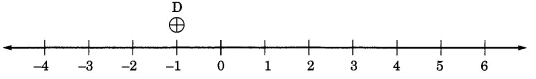(e) Here E represents the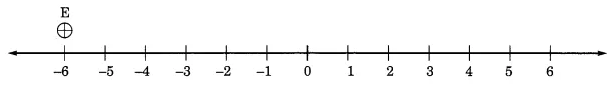(a) If point D is , then which point is ?

(b) Is point G a negative integer or a positive integer?

(c) Write integers for points B and E.

(d) Which point marked on this number line has the least value?

(e) Arrange all the points in decreasing order of value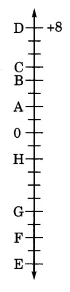(a) If D is a  then F represents  (by counting on the vertical number line)

(b) G is a negative integer because it is below than point zero (0)

(c) Integers for point B and E are  and  respectively.

(d) E has the least value of

(e) Decreasing order of all the points are-

Place                           Temperature

Siachin                        below             .................

Shimla                        below                  .................

Delhi                         above                .................

Srinagar                      below                .................

(a) Write the temperatures of these places in the form of integers in the blank column.

(b) Following is the number line representing the temperature in degree Celsius. Plot the name of the city against its temperature.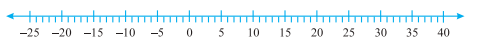(c) Which is the coolest place?

(d) Write the names of the places where temperatures are above .

(a)

 Place Temperature Integer form Siachin below -10 Shimla below -2 Ahmedabad above +30 Delhi above +20 Srinagar below -5

(b)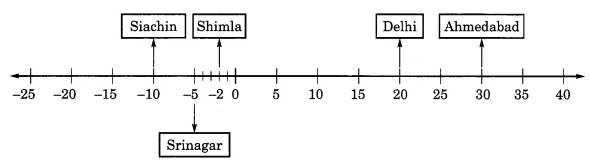(c) The coolest pace is Siachin with -10

(d) The places where the temperature is above 10  are-
(i) Delhi 20

(a)

(b)

(c)

(d)

(e)

(f)

In a number line, if an integer is greater than another integer, then it is placed on the right side of the number line. So, by this information, we can quickly identify which number is on the right side, in the given following pairs.
(a) 9 is right to the 2 ()

(b) -3 is right to the - 8 (

(c) 0 is right to the -1

(d) 10 is right to the -11

(e) 6 is right to the -6

(f) 1 is right to the -100

(a)

(b)

(c)

(d)

(a) The integers between  are;

(b) Integers between  are;

(c) Integers between  are;

(d) Integers between  are;

(b) Write four integers less than

(a)  four negative integers greater than  are;

(all negative integers wg=ho comes before -20)

(b) four integers less than are;
(integers which comes after the -10)

(a) is to the right of  on a number line.

(b) is to the right of on a number line.

(c) A smallest negative integer is .

(d) is greater than

(a) True, ( is greater than so it comes on the right side)

(b) False.  is to the left side of  on a number line

(c) False,
Correction-There is no smallest negative integer

(d) False,
Correction; is smaller than

(a) Which number will we reach if we move   numbers to the right of

(b) Which number will we reach if we move  numbers to the left of .

(c) If we are at  on the number line, in which direction should we move to reach ?

(d) If we are at on the number line, in which direction should we move to reach

(a)  if we move   numbers to the right of  we get 2.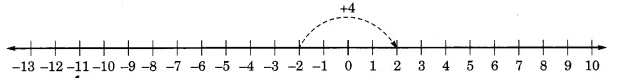(b)  if we move  numbers to the left of  we get -4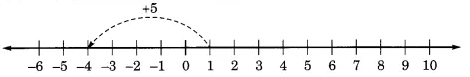(c)  If we are at  on the number line, we should move 5 number to the  left side on the number line.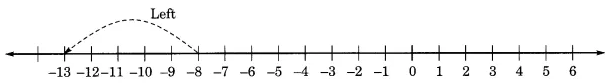(d)  If we are at on the number line, then we should move 5 number right to the  on the number line.

## NCERT solutions for class 6 maths chapter 6 Integers Exercise: 6.2

(a) more than

(b) more than

(c) less than

(d)  less than

(a) more than
The answer is 5 + 3  = 8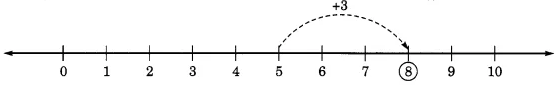(b) more than
The answer is 5 + (-5) = 0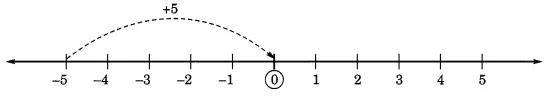(c) less than
the answer is 2 - 6 = -4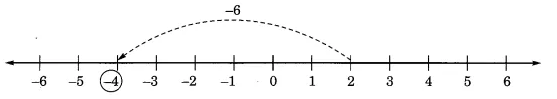(d)  less than
The answer is 3 + (-2) = 1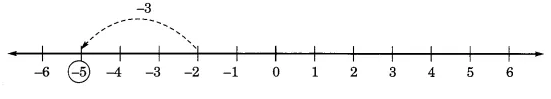(a)

(b)

(c)

(d)

(e)

(f)